Next: MHD Equations Up: Plasma Fluid Theory Previous: Normalization of the Braginskii

# Cold-Plasma Equations

Previously, we used the smallness of the magnetization parameter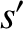to derive the cold-plasma equations: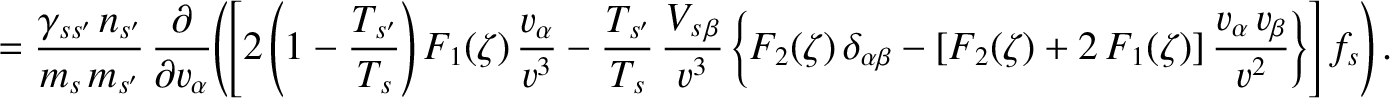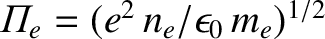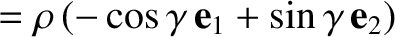(366)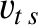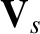(367)

and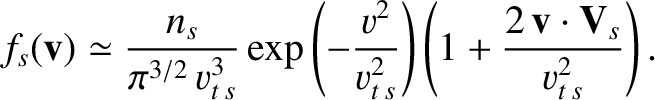(368)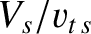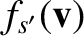(369)

Let us now use the smallness of the mass ratio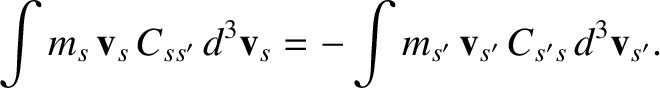to further simplify these equations. In particular, we would like to write the electron and ion fluid velocities in terms of the centre-of-mass velocity,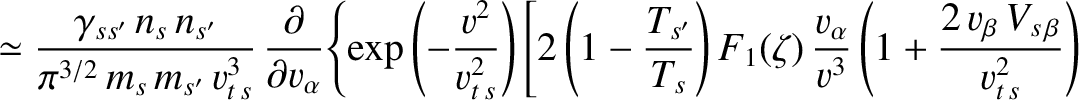(370)

and the plasma current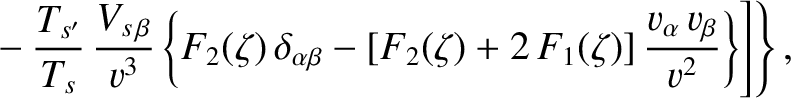(371)

where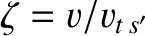. According to the ordering scheme adopted in the previous section,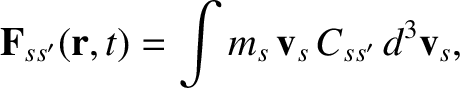in the cold-plasma limit. We shall continue to regard the mean-free-path parameter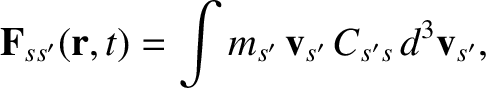as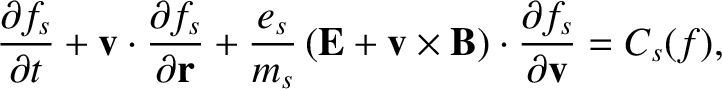.

It follows from Eqs. (370) and (371) that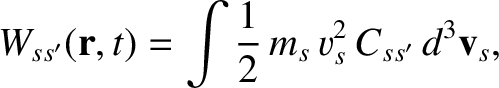(372)

and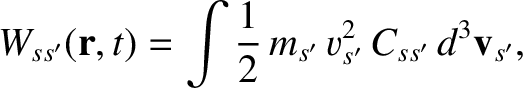(373)

Equations (366), (368), (372), and (373) yield the continuity equation: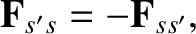(374)

where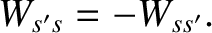. Here, use has been made of the fact that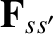in a quasi-neutral plasma.

Equations (367) and (369) can be summed to give the equation of motion: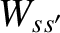(375)

Finally, Eqs. (367), (372), and (373) can be combined and to give a modified Ohm's law: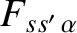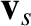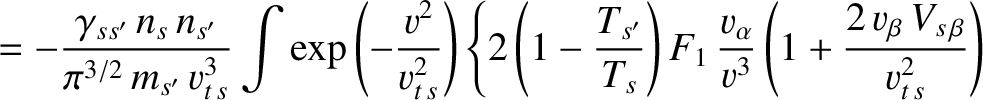(376)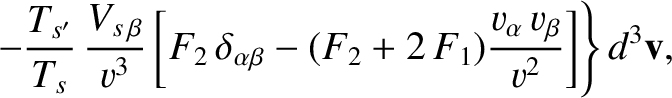The first term on the right-hand side of the above equation corresponds to resistivity, the second corresponds to the Hall effect, the third corresponds to the effect of electron inertia, and the remaining terms are usually negligible.Next: MHD Equations Up: Plasma Fluid Theory Previous: Normalization of the Braginskii
Richard Fitzpatrick 2011-03-31